# Beamforming

## 1. 阵列简介

### 1.1 均匀线阵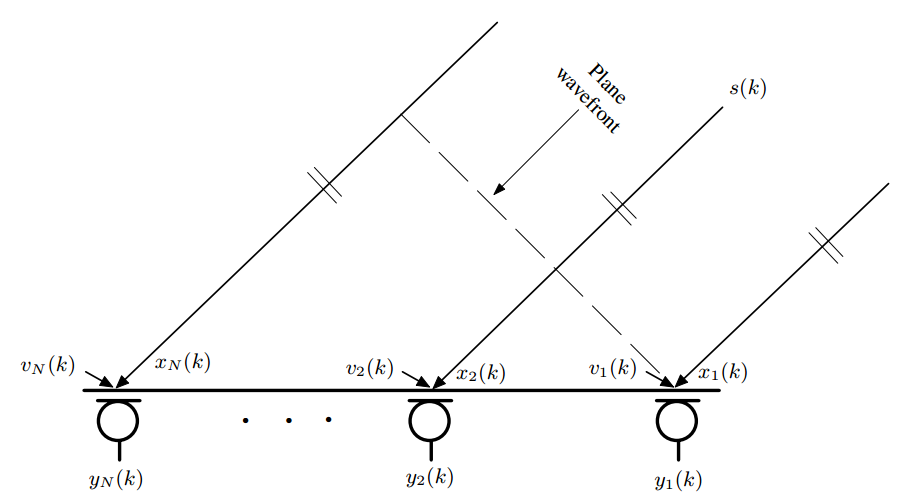假设0、1、…M-1阵元的均匀线阵中，参考阵元接收的信号为$s\left(t\right)$$s(t)$，则第$m$$m$个阵元接收到的信号为$s\left(t-{\tau }_{m}\right)$$s(t-\tau_m)$，傅里叶变换如下

${S}_{m}\left(\omega \right)={\int }_{-\mathrm{\infty }}^{+\mathrm{\infty }}{s}_{m}\left(t\right){e}^{-j\omega t}dt$$S_m(\omega)=\int_{-\infty }^{+\infty} s_m(t)e^{-j\omega t}dt$

$={\int }_{-\mathrm{\infty }}^{+\mathrm{\infty }}s\left(t-{\tau }_{m}\right){e}^{-j\omega t}dt$$=\int_{-\infty }^{+\infty} s(t-\tau_m)e^{-j\omega t}dt$

$=S\left(\omega \right){e}^{-j\omega {\tau }_{m}}$$=S(\omega)e^{-j\omega\tau_m}$

$X\left(\omega \right)=S\left(\omega \right)\left[1 {e}^{-j\omega {\tau }_{１}} {e}^{-j\omega {\tau }_{２}} \dots {e}^{-j\omega {\tau }_{M-1}}\right]$$X(\omega)=S(\omega)[1 e^{-j\omega\tau_１} e^{-j\omega\tau_２} … e^{-j\omega\tau_{M-1}} ]$

$\stackrel{\to }{a}=\left[1 {e}^{-j\omega {\tau }_{１}} {e}^{-j\omega {\tau }_{２}} \dots {e}^{-j\omega {\tau }_{M-1}}\right]$$\vec{a}=[1 e^{-j\omega\tau_１} e^{-j\omega\tau_２} … e^{-j\omega\tau_{M-1}} ]$

在delauy-and-sum beamformer中，每个阵元的权值即为该阵元相对参考阵元需要补偿的相位延迟，因此权值即为阵列流形的共轭转置
根据上面的分析，写出窄带线性阵列的delay-and-sum波束图代码如下

#### delay-and-sum beamformer

M=8;                 % 阵元数
c=340;                         % 声速
f = 2125;                      % 信号频率
d = 0.08;                      % 阵元间距
theta=linspace(-pi/2,pi/2,200);% 入射信号角度范围
theta0=30*pi/180;                      % 注视方向
w=exp(1j*2*pi*f*sin(theta0)*[0:M-1]'*d/c);       % 导向向量
p = zeros(length(1:length(theta)),1);
for  j=1:length(theta)                                     % 角度扫描
a=exp(-1j*2*pi*f*sin(theta(j))*[0:M-1]'*d/c);% 入射信号方向向量
p(j)=sum(w.*a)/M;                                        % 延时-求和
end
% 画图
figure;
plot(theta/pi*180,abs(p)),grid on
xlabel('degree')
ylabel('amplitude')
title('8阵元均匀线阵方向图')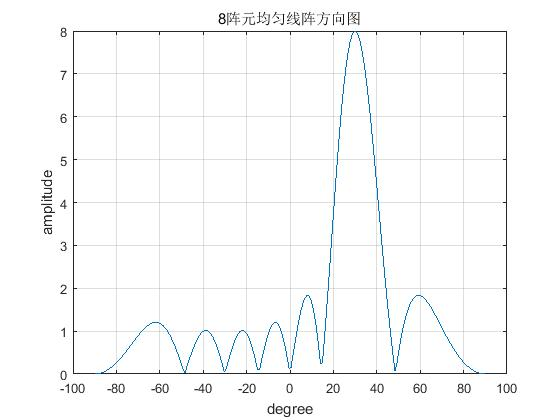### 1.2 均匀圆阵

跟上面的分析相同，关键地方就是写出阵列流形向量和权值向量
假设$M$$M$阵元均匀圆阵，半径为$R$$R$，阵元在$x-y$$x-y$平面上，则各个阵元相对$x$$x$轴的夹角${\gamma }_{m}=2\pi m/M$$\gamma_m=2\pi m/M$，均匀圆阵中，一般以圆心为参考，要写出阵列方向向量，就是要计算当单位平面波以夹角$\left(\varphi ，\theta \right)$$(\phi，\theta)$入射时，各阵元相对圆心接收到的信号时间差。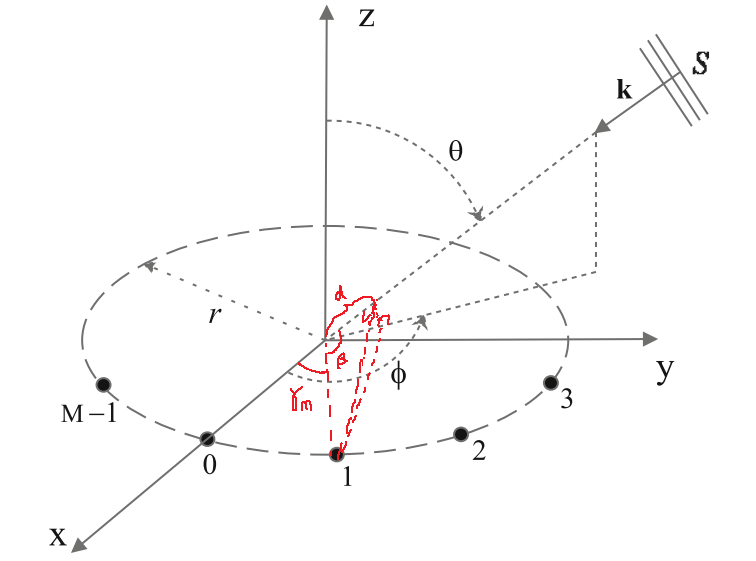计算上图1

$cos\left(\beta \right)=cos\left(\varphi -{\gamma }_{m}\right)sin\left(\theta \right)$$cos(\beta)=cos(\phi-\gamma_m)sin(\theta)$

${\tau }_{m}=Ｒcos\left(\varphi -{\gamma }_{m}\right)sin\left(\theta \right)／ｃ$$\tau_m=Ｒcos(\phi-\gamma_m)sin(\theta)／ｃ$

$\vec{a}=\begin{bmatrix}e^{-j\omega Ｒcos(\phi-\gamma_0)sin(\theta)/c} \ e^{-j\omega Ｒcos(\phi-\gamma_1)sin(\theta)/c} \ … \ e^{-j\omega Ｒcos(\phi-\gamma_{M-1})sin(\theta)/c} \end{bmatrix}$

M=8;                 % 阵元数
c=340;                         % 声速
f = 2125;                      % 信号频率
r = 0.08;                      % 阵元间距
phi=linspace(0,2*pi,360);% 入射信号角度范围

theta = 80*pi/180; %固定一个俯仰角
gamma = (0:360/M:360-360/M)*pi/180;%麦克风位置

tao = r*sin(theta)*cos(angle(1)-gamma)/c;     %方位角 0 < angle <360

phi0=60*pi/180;                      % 注视方向
w=exp(1j*2*pi*f*r*cos(phi0-gamma)*sin(theta)/c);       % 导向向量
p = zeros(length(1:length(phi)),1);
for  j=1:length(phi)                                     % 角度扫描
a=exp(-1j*2*pi*f*r*cos(phi(j)-gamma)*sin(theta)/c);% 入射信号方向向量
p(j)=sum(w.*a)/M;                                        % 延时-求和
end
% 画图
figure;
plot(phi/pi*180,abs(p)),grid on
xlabel('degree')
ylabel('amplitude')
title('均匀圆阵方向图')

%画极坐标图
figure,polarplot(phi,abs(p))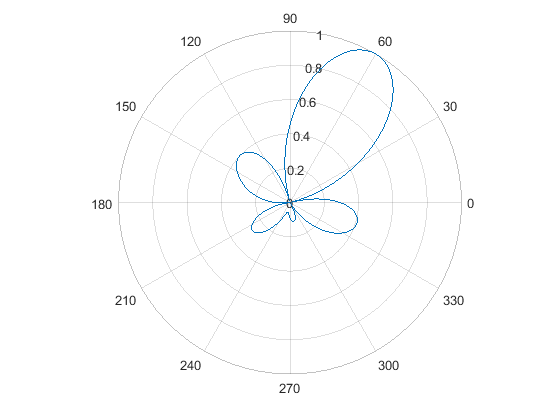M=8;                 % 阵元数
c=340;                         % 声速
f = 2125;                      % 信号频率
r = 0.08;                      % 阵元间距
phi=linspace(0,2*pi,360);% 入射信号方位角范围

theta = linspace(0,pi/2,90);% 入射信号俯仰角范围

gamma = (0:360/M:360-360/M)*pi/180;%麦克风位置

phi0=130*pi/180;                      % 注视方向
theta0 = 80*pi/180;

w=exp(1j*2*pi*f*r*cos(phi0-gamma)*sin(theta0)/c);       % 导向向量
p = zeros(length(1:length(phi)),length(theta));
for i = 1:length(theta)
for  j=1:length(phi)                                     % 角度扫描
a=exp(-1j*2*pi*f*r*cos(phi(j)-gamma)*sin(theta(i))/c);% 入射信号方向向量
p(j,i)=sum(a.*w)/M;                                        % 延时-求和
end
end
% 画图

figure,mesh(abs(p))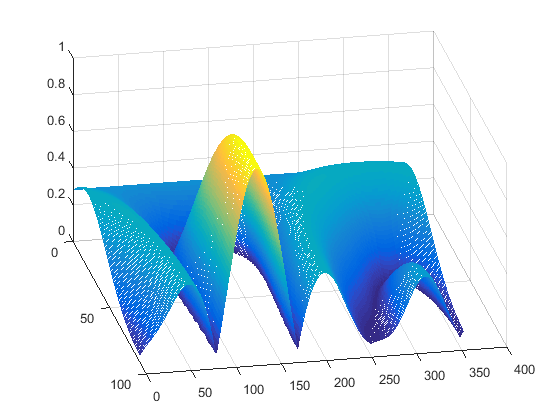## 2. 宽带波束形成

### 2.1 时域处理

(TDLs)，框图如下，一些时域LCMV、MVDR都属于这种框架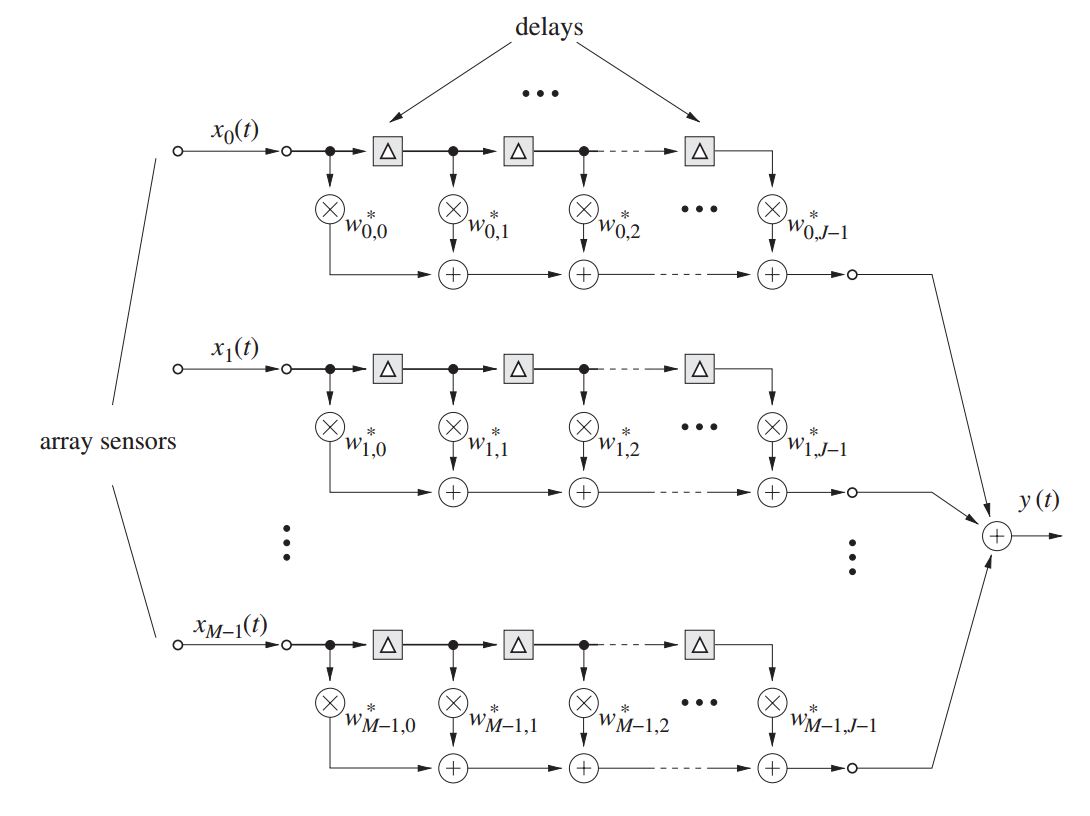### 2.2 频域处理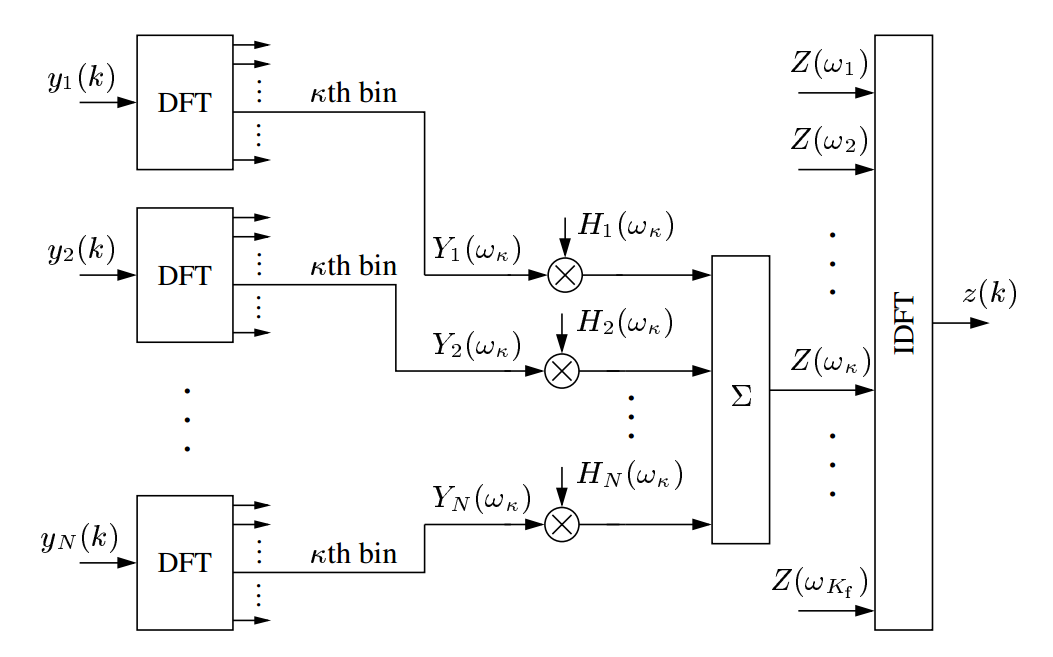1. Design of robust high-order superdirectivity for circular arrays with sensor gain and phase errors。